# Optimization Methods Optimization models Single x Multiobjective models

• Slides: 66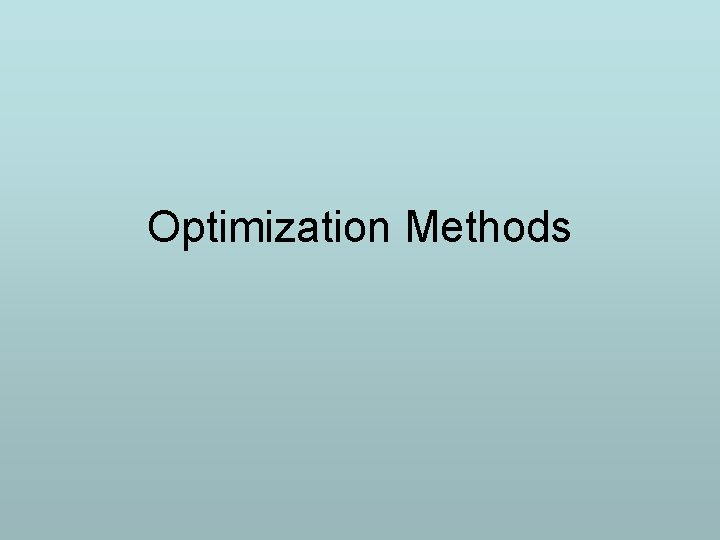Optimization Methods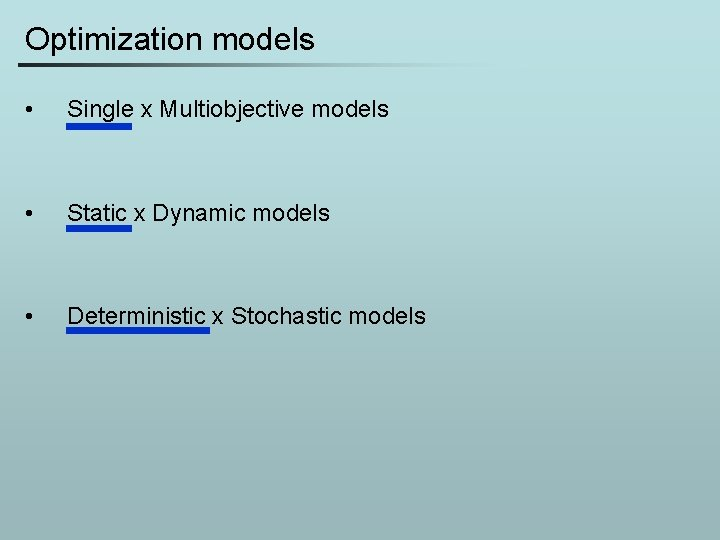Optimization models • Single x Multiobjective models • Static x Dynamic models • Deterministic x Stochastic models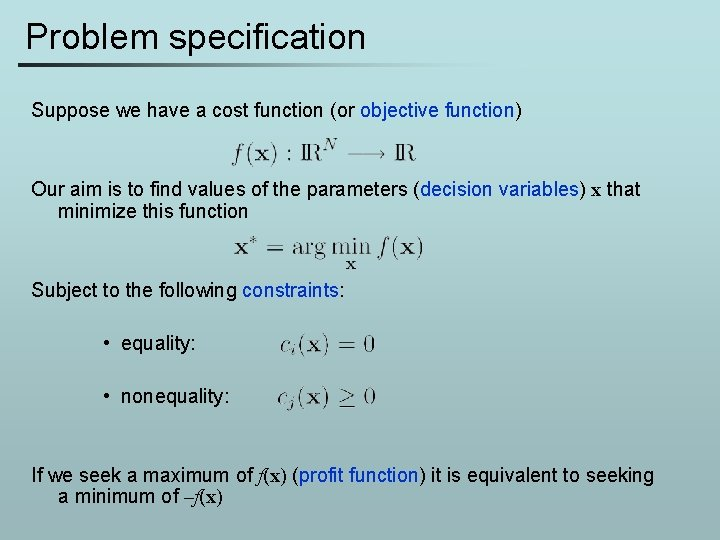Problem specification Suppose we have a cost function (or objective function) Our aim is to find values of the parameters (decision variables) x that minimize this function Subject to the following constraints: • equality: • nonequality: If we seek a maximum of f(x) (profit function) it is equivalent to seeking a minimum of –f(x)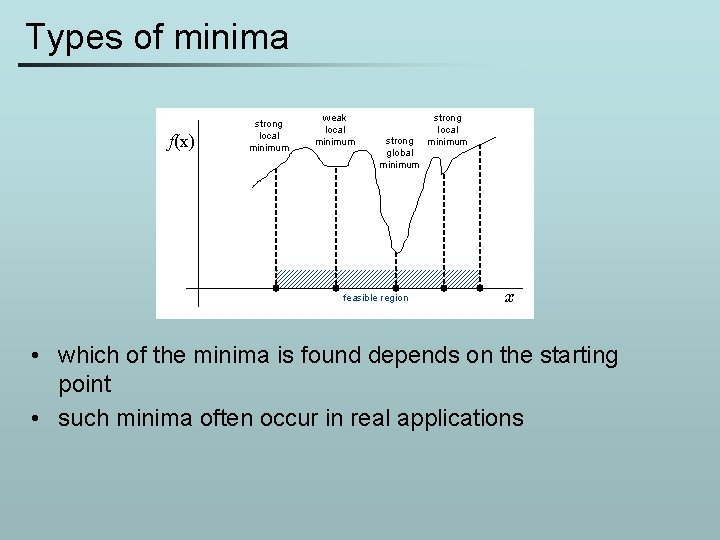Types of minima f(x) strong local minimum weak local minimum strong global minimum feasible region strong local minimum x • which of the minima is found depends on the starting point • such minima often occur in real applications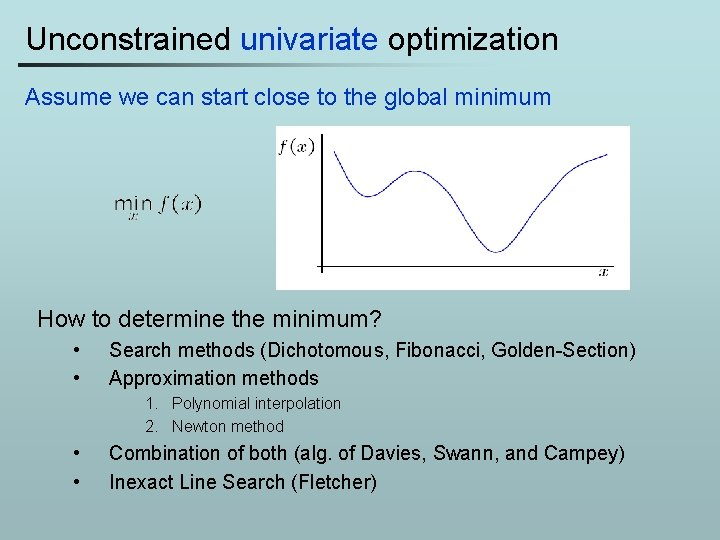Unconstrained univariate optimization Assume we can start close to the global minimum How to determine the minimum? • • Search methods (Dichotomous, Fibonacci, Golden-Section) Approximation methods 1. Polynomial interpolation 2. Newton method • • Combination of both (alg. of Davies, Swann, and Campey) Inexact Line Search (Fletcher)1 D function As an example consider the function (assume we do not know the actual function expression from now on)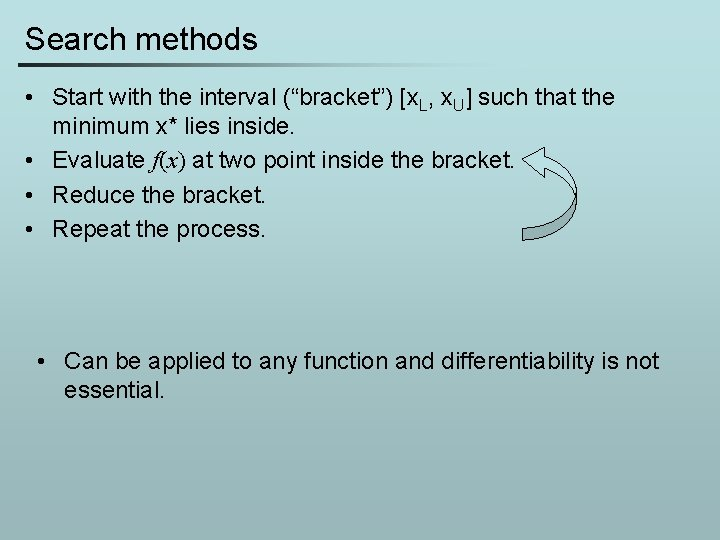Search methods • Start with the interval (“bracket”) [x. L, x. U] such that the minimum x* lies inside. • Evaluate f(x) at two point inside the bracket. • Reduce the bracket. • Repeat the process. • Can be applied to any function and differentiability is not essential.Search methods x. U x. L x. U Dichotomous Fibonacci: 1 1 2 3 5 8… Ik+5 Ik+4 Ik+3 Ik+2 Ik+1 Ik 1 2 3 x. L 5 8 x. U Golden-Section Search divides intervals by K = 1. 6180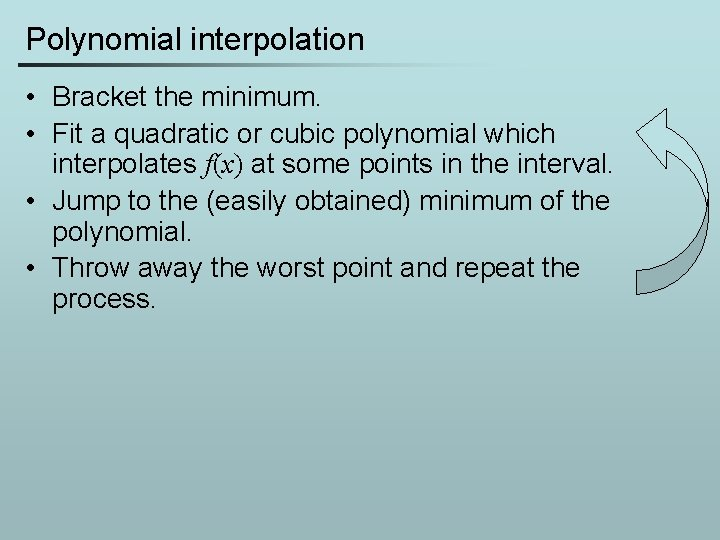Polynomial interpolation • Bracket the minimum. • Fit a quadratic or cubic polynomial which interpolates f(x) at some points in the interval. • Jump to the (easily obtained) minimum of the polynomial. • Throw away the worst point and repeat the process.Polynomial interpolation • Quadratic interpolation using 3 points, 2 iterations • Other methods to interpolate? – 2 points and one gradient – Cubic interpolation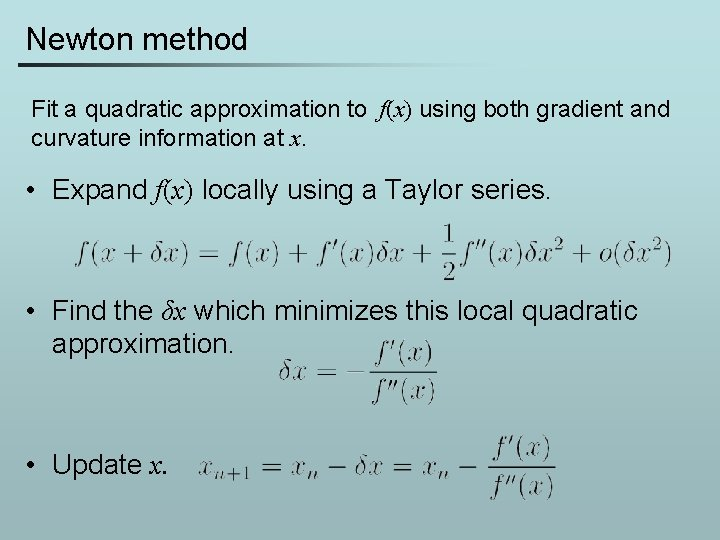Newton method Fit a quadratic approximation to f(x) using both gradient and curvature information at x. • Expand f(x) locally using a Taylor series. • Find the δx which minimizes this local quadratic approximation. • Update x.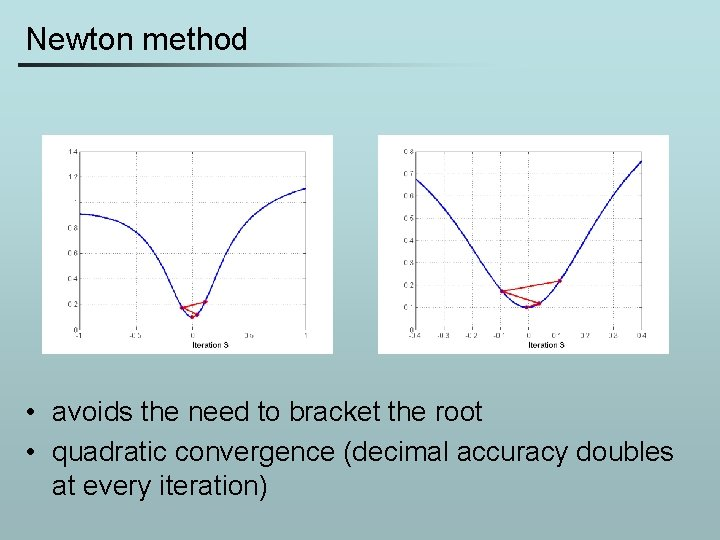Newton method • avoids the need to bracket the root • quadratic convergence (decimal accuracy doubles at every iteration)Newton method • Global convergence of Newton’s method is poor. • Often fails if the starting point is too far from the minimum. • in practice, must be used with a globalization strategy which reduces the step length until function decrease is assured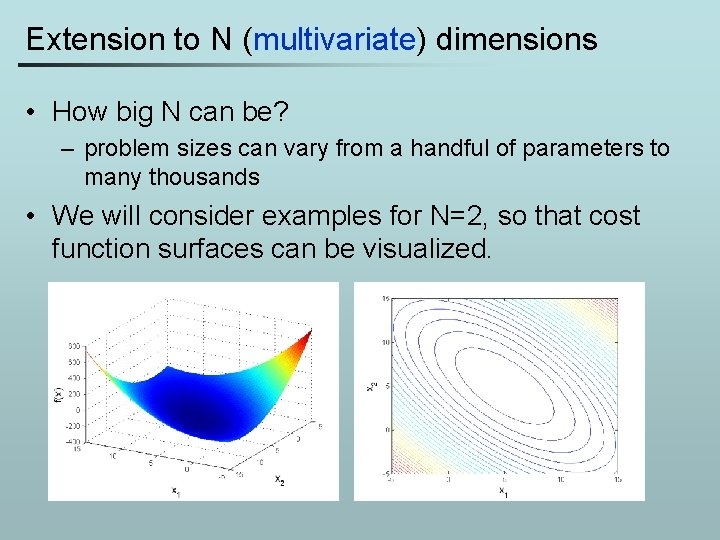Extension to N (multivariate) dimensions • How big N can be? – problem sizes can vary from a handful of parameters to many thousands • We will consider examples for N=2, so that cost function surfaces can be visualized.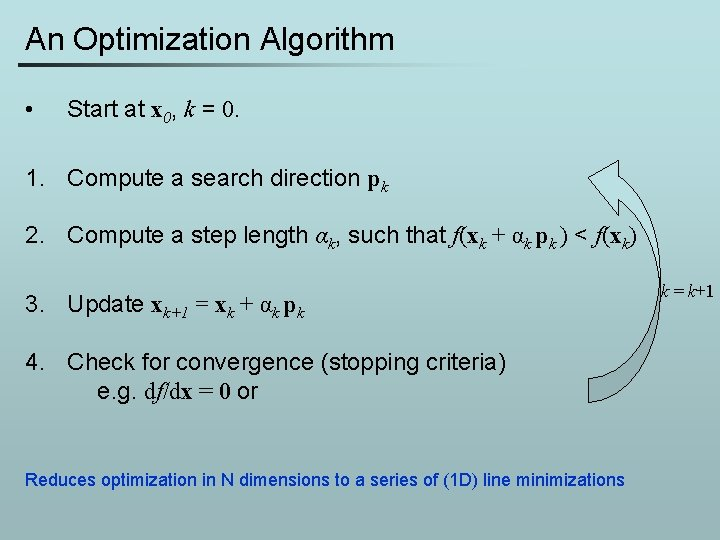An Optimization Algorithm • Start at x 0, k = 0. 1. Compute a search direction pk 2. Compute a step length αk, such that f(xk + αk pk ) < f(xk) 3. Update xk+1 = xk + αk pk 4. Check for convergence (stopping criteria) e. g. df/dx = 0 or Reduces optimization in N dimensions to a series of (1 D) line minimizations k = k+1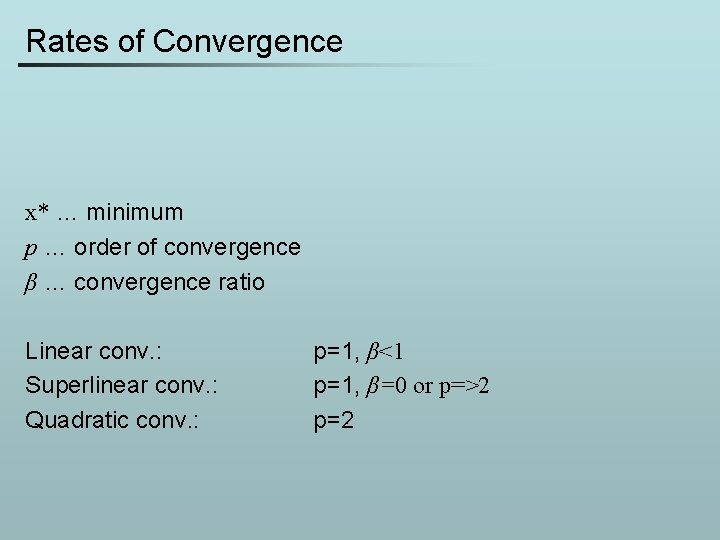Rates of Convergence x* … minimum p … order of convergence β … convergence ratio Linear conv. : Superlinear conv. : Quadratic conv. : p=1, β<1 p=1, β=0 or p=>2 p=2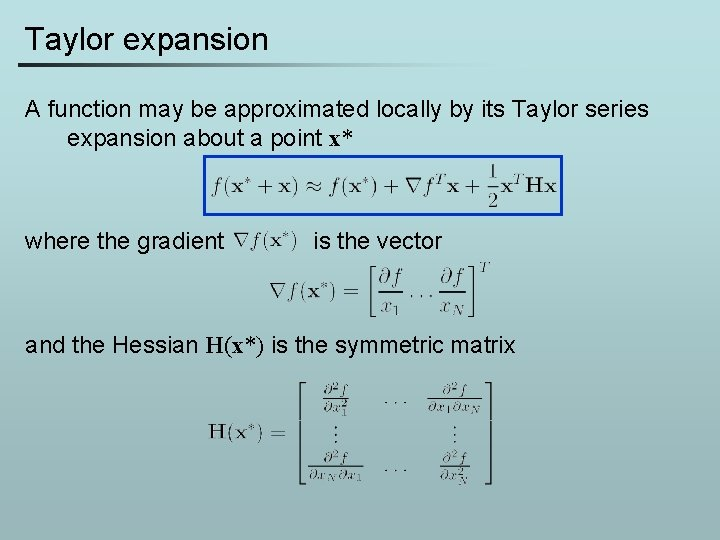Taylor expansion A function may be approximated locally by its Taylor series expansion about a point x* where the gradient is the vector and the Hessian H(x*) is the symmetric matrixQuadratic functions • • The vector g and the Hessian H are constant. Second order approximation of any function by the Taylor expansion is a quadratic function. We will assume only quadratic functions for a while.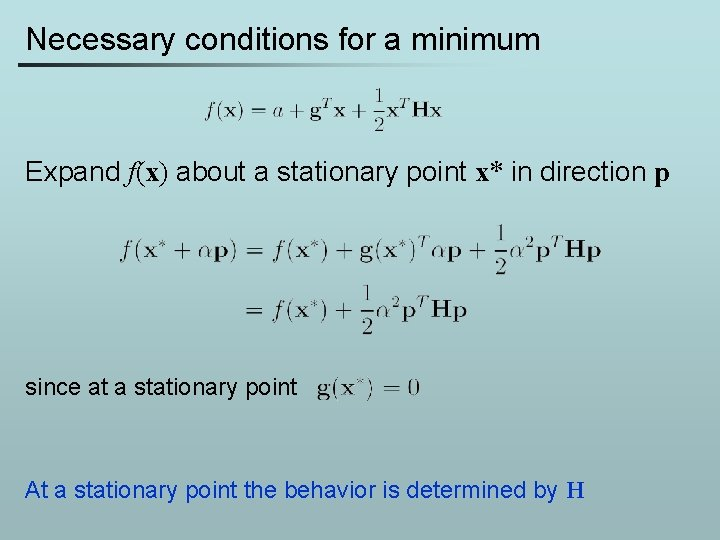Necessary conditions for a minimum Expand f(x) about a stationary point x* in direction p since at a stationary point At a stationary point the behavior is determined by H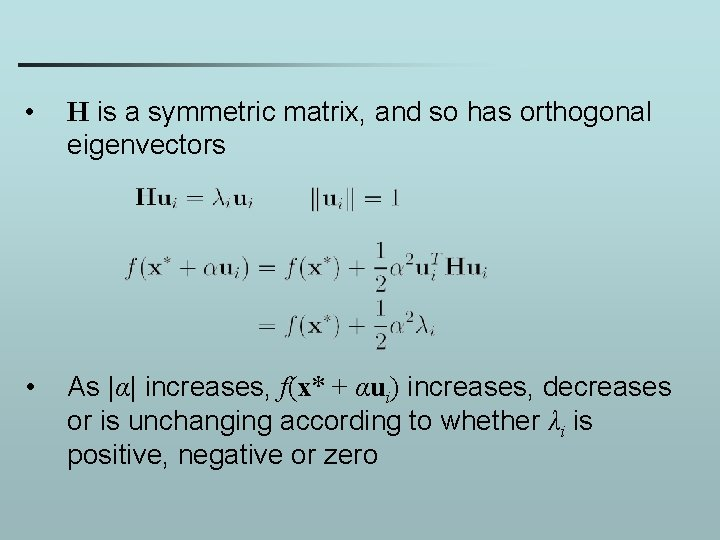• H is a symmetric matrix, and so has orthogonal eigenvectors • As |α| increases, f(x* + αui) increases, decreases or is unchanging according to whether λi is positive, negative or zero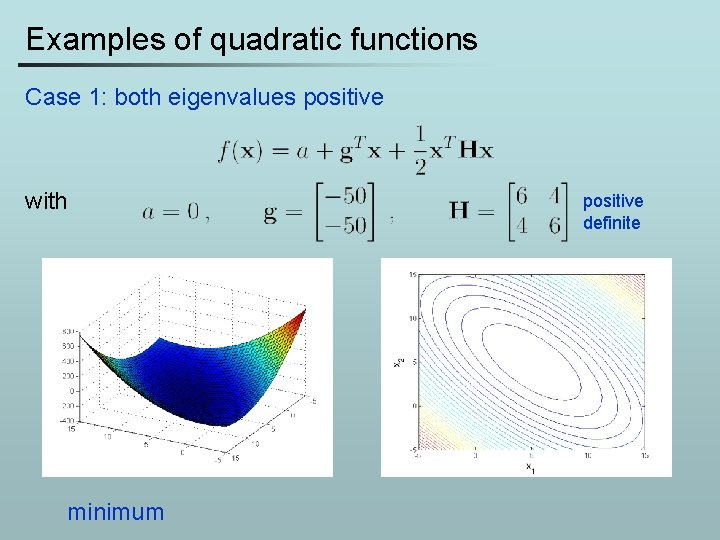Examples of quadratic functions Case 1: both eigenvalues positive with minimum positive definite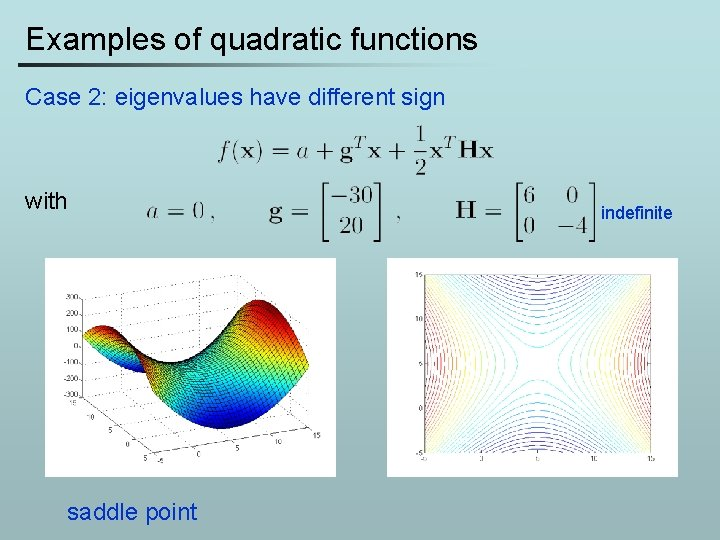Examples of quadratic functions Case 2: eigenvalues have different sign with saddle point indefinite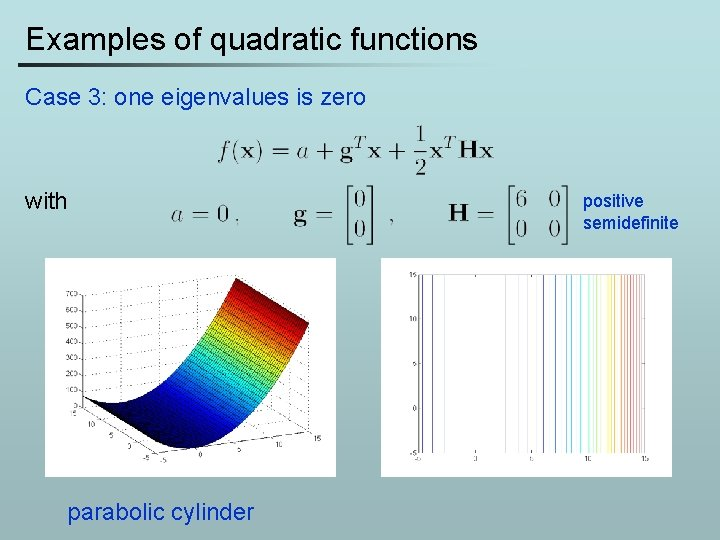Examples of quadratic functions Case 3: one eigenvalues is zero with parabolic cylinder positive semidefinite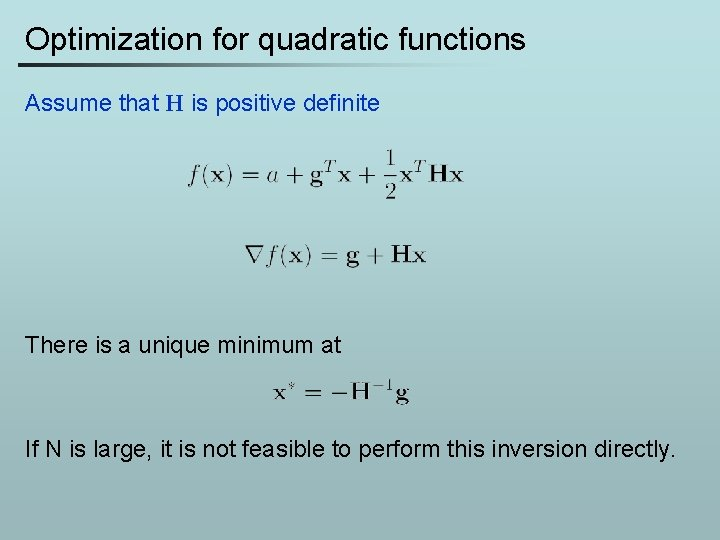Optimization for quadratic functions Assume that H is positive definite There is a unique minimum at If N is large, it is not feasible to perform this inversion directly.Steepest descent • Basic principle is to minimize the N-dimensional function by a series of 1 D line-minimizations: • The steepest descent method chooses pk to be parallel to the gradient • Step-size αk is chosen to minimize f(xk + αkpk). For quadratic forms there is a closed form solution: Prove it!Steepest descent • The gradient is everywhere perpendicular to the contour lines. • After each line minimization the new gradient is always orthogonal to the previous step direction (true of any line minimization). • Consequently, the iterates tend to zig-zag down the valley in a very inefficient mannerConjugate gradient • Each pk is chosen to be conjugate to all previous search directions with respect to the Hessian H: • The resulting search directions are mutually linearly independent. Prove it! • Remarkably, pk can be chosen using only knowledge of pk , and -1,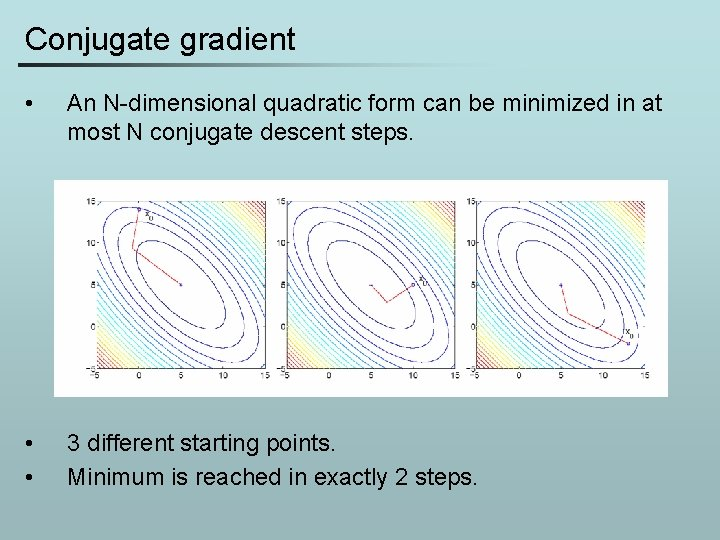Conjugate gradient • An N-dimensional quadratic form can be minimized in at most N conjugate descent steps. • • 3 different starting points. Minimum is reached in exactly 2 steps.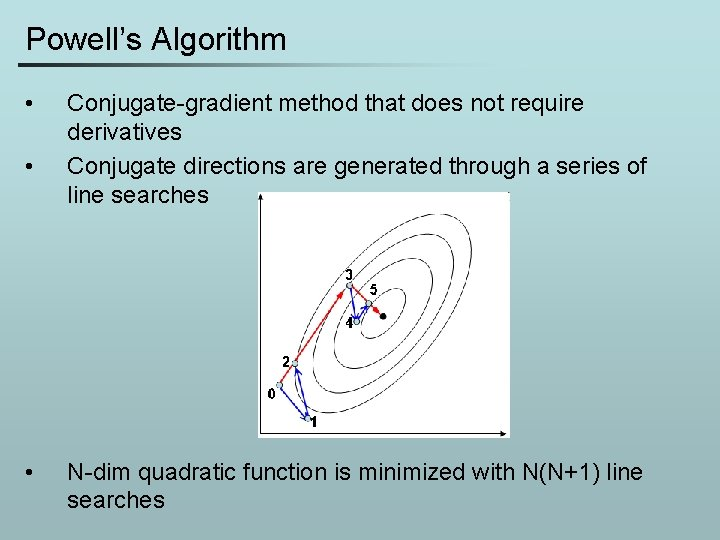Powell’s Algorithm • • • Conjugate-gradient method that does not require derivatives Conjugate directions are generated through a series of line searches N-dim quadratic function is minimized with N(N+1) line searches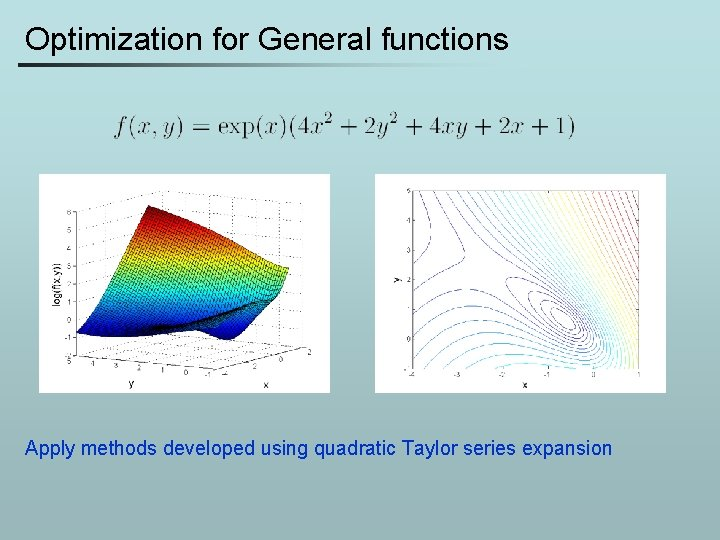Optimization for General functions Apply methods developed using quadratic Taylor series expansion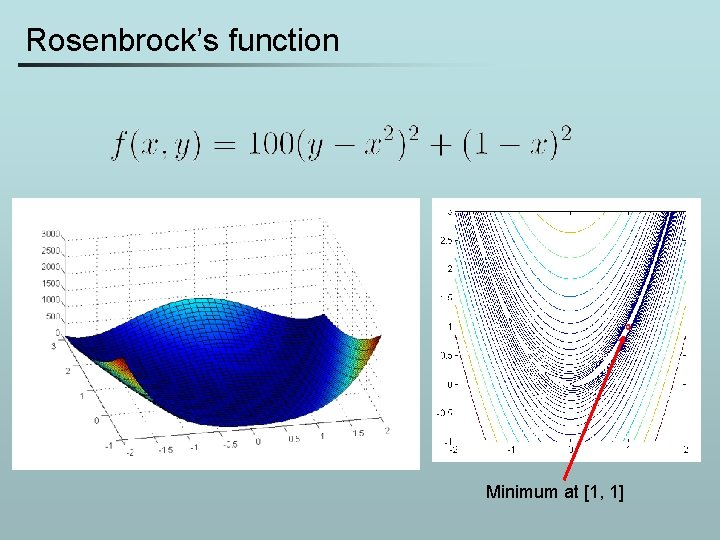Rosenbrock’s function Minimum at [1, 1]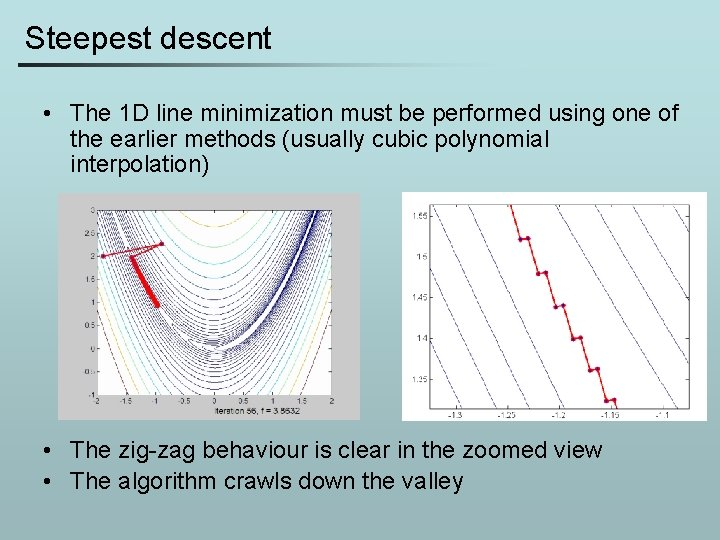Steepest descent • The 1 D line minimization must be performed using one of the earlier methods (usually cubic polynomial interpolation) • The zig-zag behaviour is clear in the zoomed view • The algorithm crawls down the valleyConjugate gradient • Again, an explicit line minimization must be used at every step • The algorithm converges in 98 iterations • Far superior to steepest descentNewton method Expand f(x) by its Taylor series about the point xk where the gradient is the vector and the Hessian is the symmetric matrix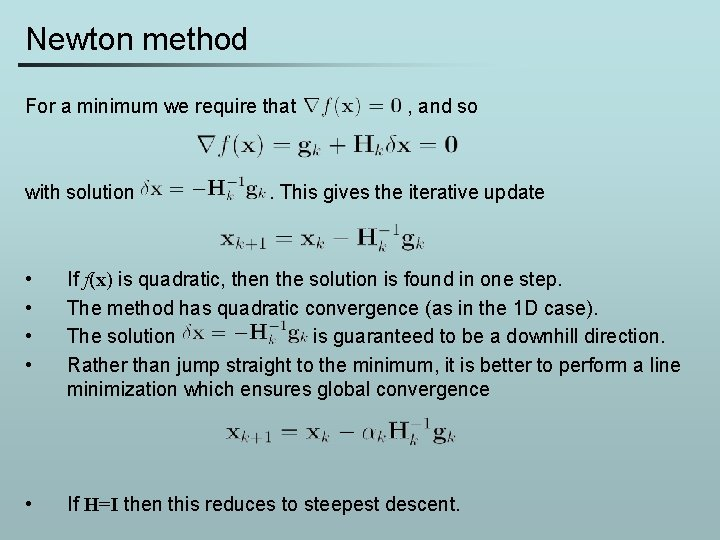Newton method For a minimum we require that with solution , and so . This gives the iterative update • • If f(x) is quadratic, then the solution is found in one step. The method has quadratic convergence (as in the 1 D case). The solution is guaranteed to be a downhill direction. Rather than jump straight to the minimum, it is better to perform a line minimization which ensures global convergence • If H=I then this reduces to steepest descent.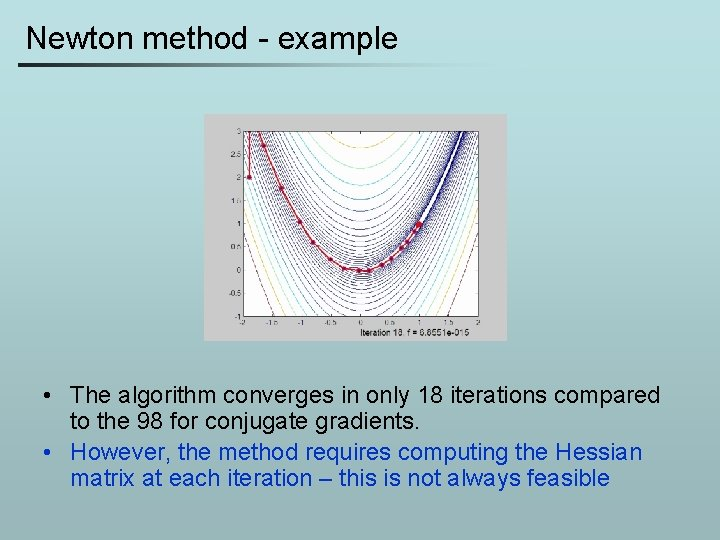Newton method - example • The algorithm converges in only 18 iterations compared to the 98 for conjugate gradients. • However, the method requires computing the Hessian matrix at each iteration – this is not always feasibleQuasi-Newton methods • • If the problem size is large and the Hessian matrix is dense then it may be infeasible/inconvenient to compute it directly. Quasi-Newton methods avoid this problem by keeping a “rolling estimate” of H(x), updated at each iteration using new gradient information. Common schemes are due to Broyden, Goldfarb, Fletcher and Shanno (BFGS), and also Davidson, Fletcher and Powell (DFP). The idea is based on the fact that for quadratic functions holds and by accumulating gk’s and xk’s we can calculate H.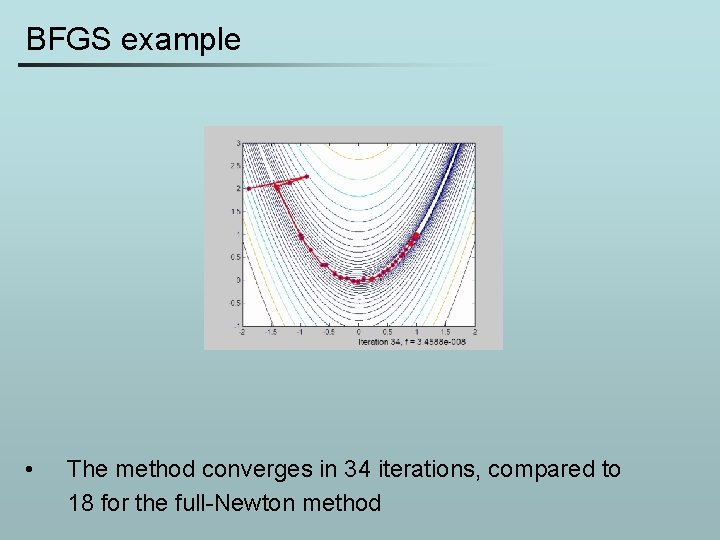BFGS example • The method converges in 34 iterations, compared to 18 for the full-Newton methodNon-linear least squares • It is very common in applications for a cost function f(x) to be the sum of a large number of squared residuals • If each residual depends non-linearly on the parameters x then the minimization of f(x) is a non-linear least squares problem.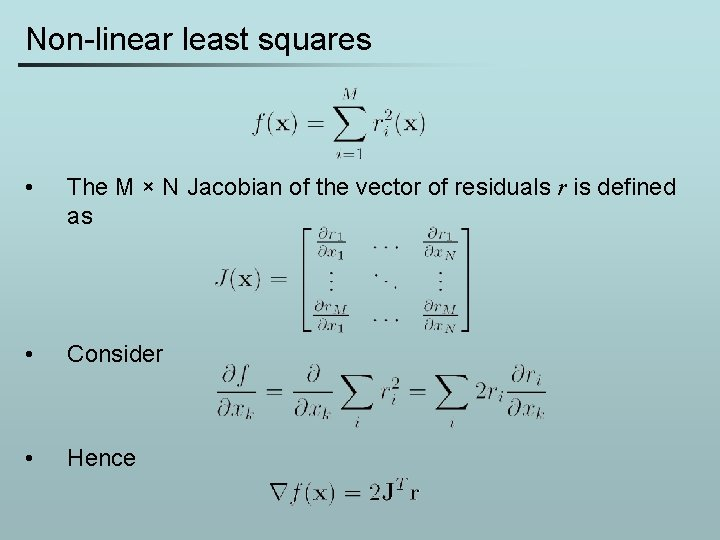Non-linear least squares • The M × N Jacobian of the vector of residuals r is defined as • Consider • HenceNon-linear least squares • For the Hessian holds Gauss-Newton approximation • • • Note that the second-order term in the Hessian is multiplied by the residuals ri. In most problems, the residuals will typically be small. Also, at the minimum, the residuals will typically be distributed with mean = 0. • • For these reasons, the second-order term is often ignored. Hence, explicit computation of the full Hessian can again be avoided.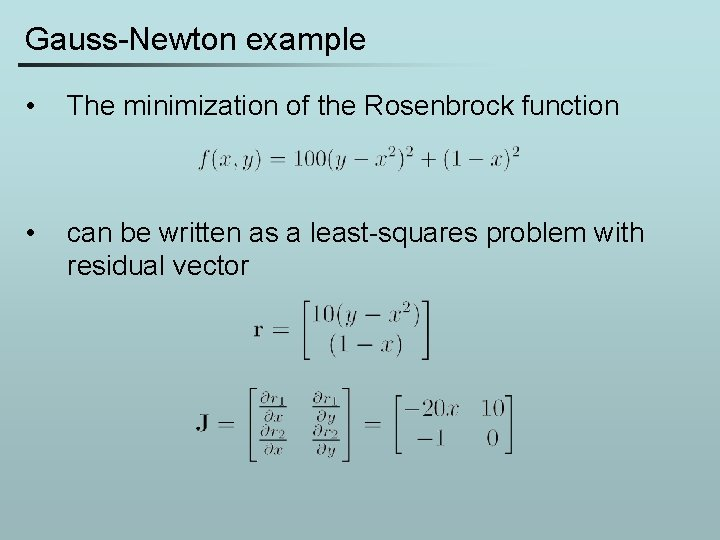Gauss-Newton example • The minimization of the Rosenbrock function • can be written as a least-squares problem with residual vectorGauss-Newton example • minimization with the Gauss-Newton approximation with line search takes only 11 iterations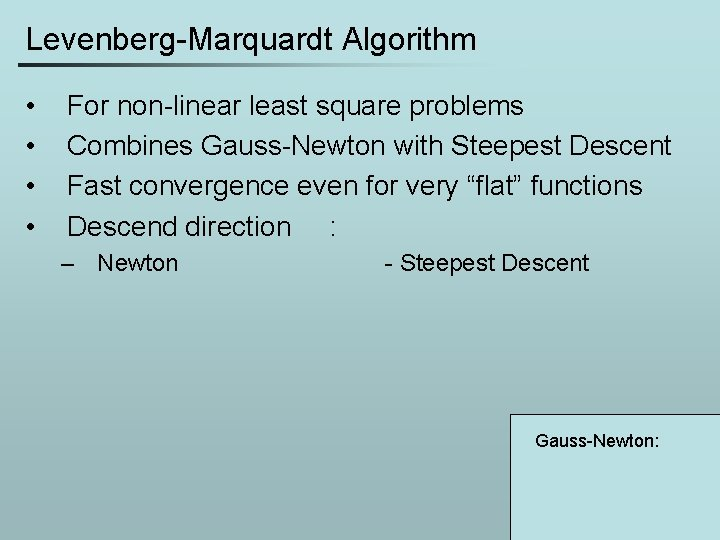Levenberg-Marquardt Algorithm • • For non-linear least square problems Combines Gauss-Newton with Steepest Descent Fast convergence even for very “flat” functions Descend direction : – Newton - Steepest Descent Gauss-Newton:Comparison CG Quasi-Newton Gauss-Newton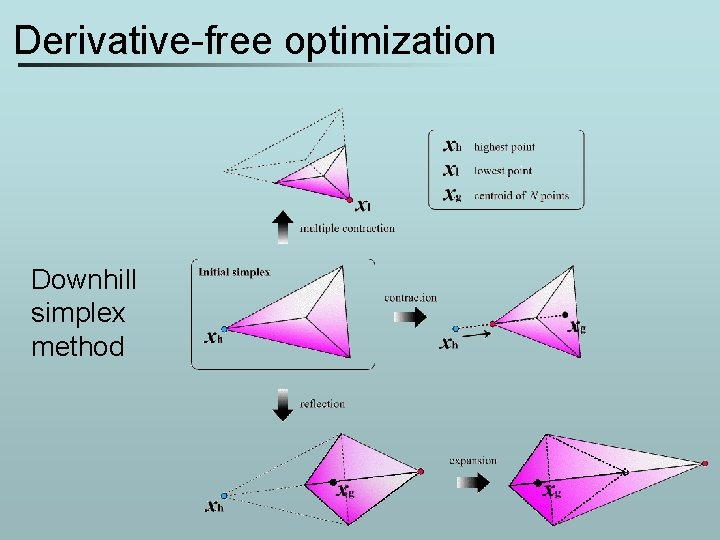Derivative-free optimization Downhill simplex method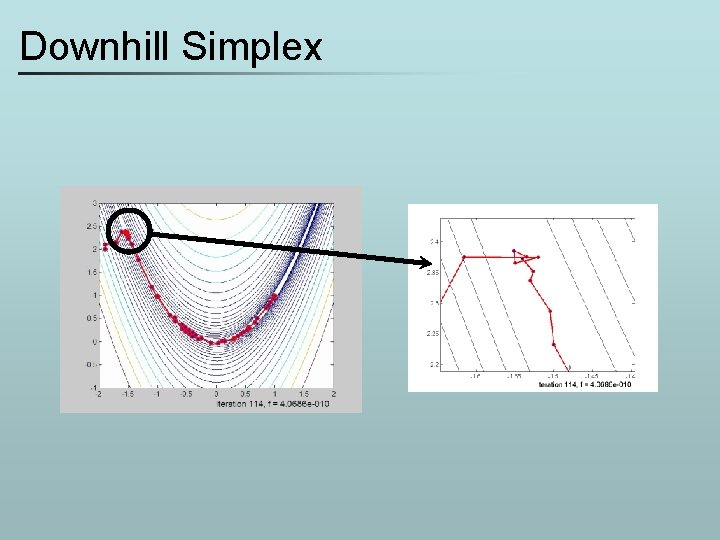Downhill SimplexComparison CG Quasi-Newton Downhill Simplex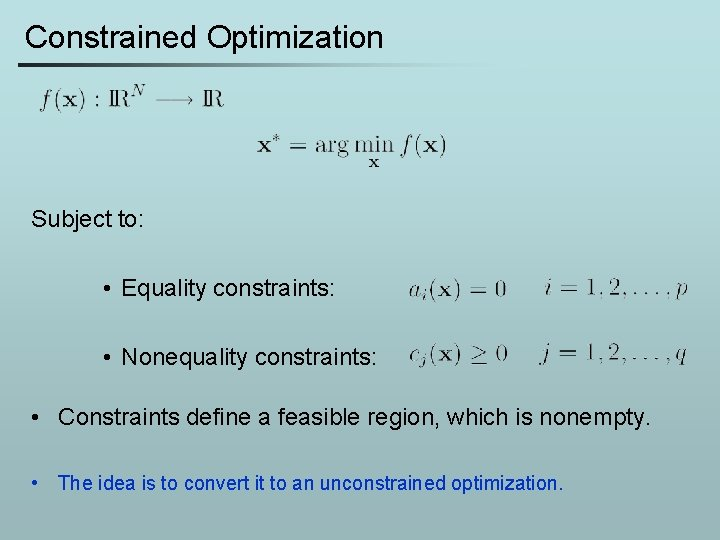Constrained Optimization Subject to: • Equality constraints: • Nonequality constraints: • Constraints define a feasible region, which is nonempty. • The idea is to convert it to an unconstrained optimization.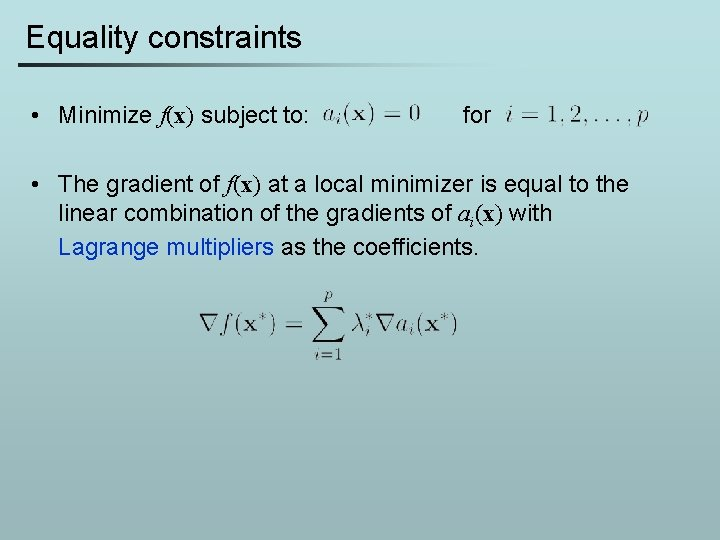Equality constraints • Minimize f(x) subject to: for • The gradient of f(x) at a local minimizer is equal to the linear combination of the gradients of ai(x) with Lagrange multipliers as the coefficients.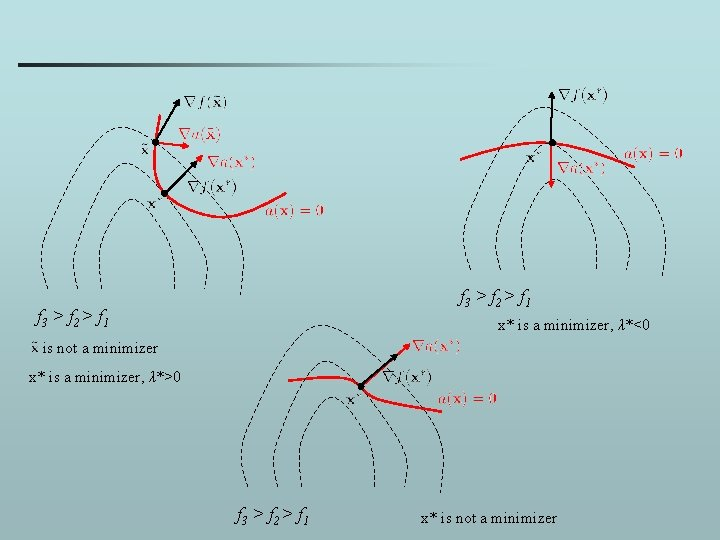f 3 > f 2 > f 1 x* is a minimizer, λ*<0 is not a minimizer x* is a minimizer, λ*>0 f 3 > f 2 > f 1 x* is not a minimizer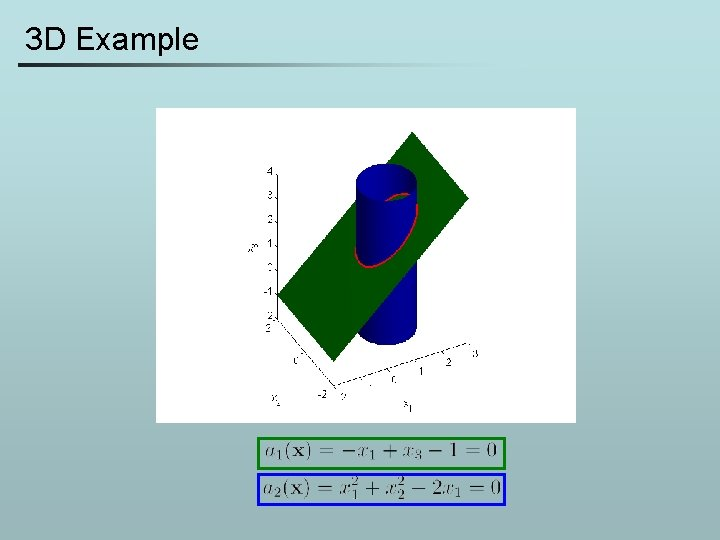3 D Example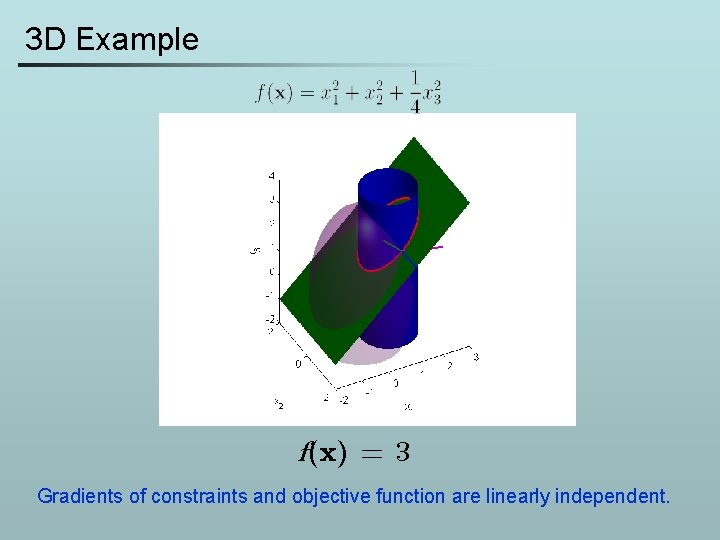3 D Example f(x) = 3 Gradients of constraints and objective function are linearly independent.3 D Example f(x) = 1 Gradients of constraints and objective function are linearly dependent.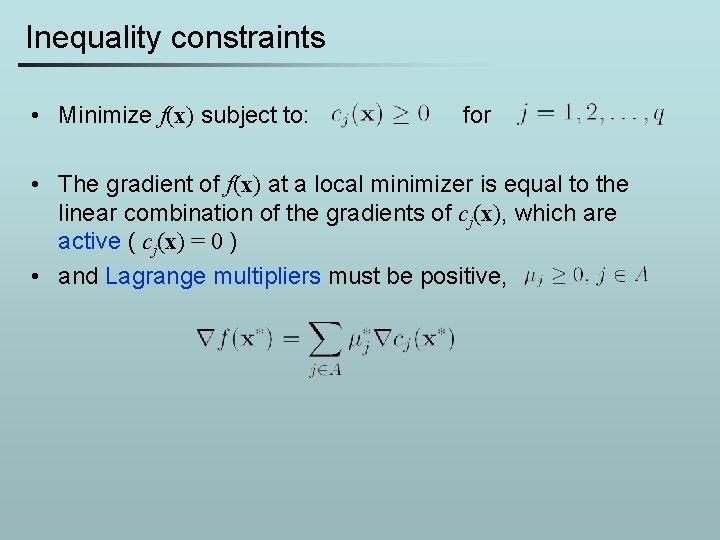Inequality constraints • Minimize f(x) subject to: for • The gradient of f(x) at a local minimizer is equal to the linear combination of the gradients of cj(x), which are active ( cj(x) = 0 ) • and Lagrange multipliers must be positive,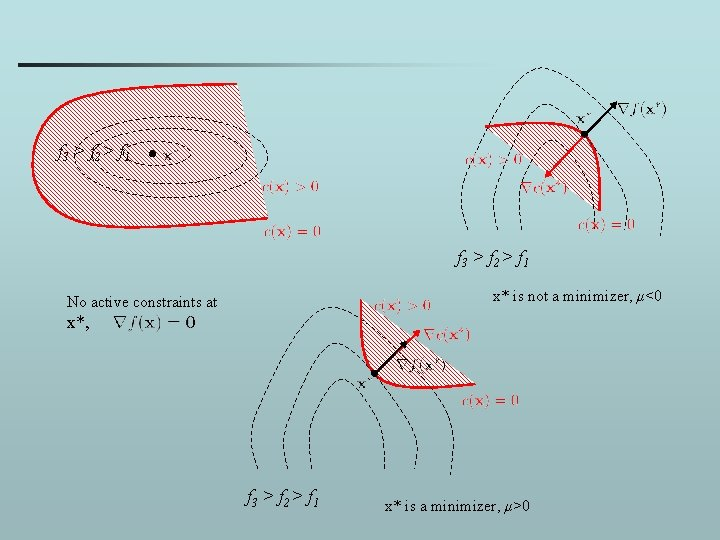f 3 > f 2 > f 1 x* is not a minimizer, μ<0 No active constraints at x*, f 3 > f 2 > f 1 x* is a minimizer, μ>0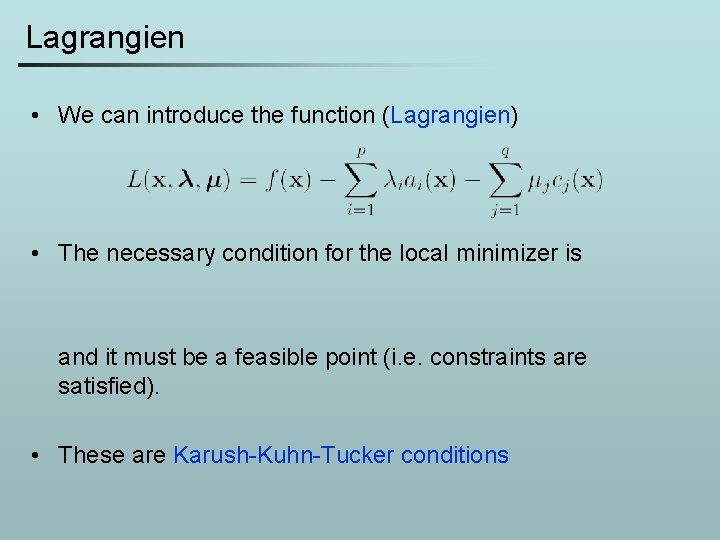Lagrangien • We can introduce the function (Lagrangien) • The necessary condition for the local minimizer is and it must be a feasible point (i. e. constraints are satisfied). • These are Karush-Kuhn-Tucker conditionsQuadratic Programming (QP) • Like in the unconstrained case, it is important to study quadratic functions. Why? • Because general nonlinear problems are solved as a sequence of minimizations of their quadratic approximations. • QP with constraints Minimize subject to linear constraints. • H is symmetric and positive semidefinite.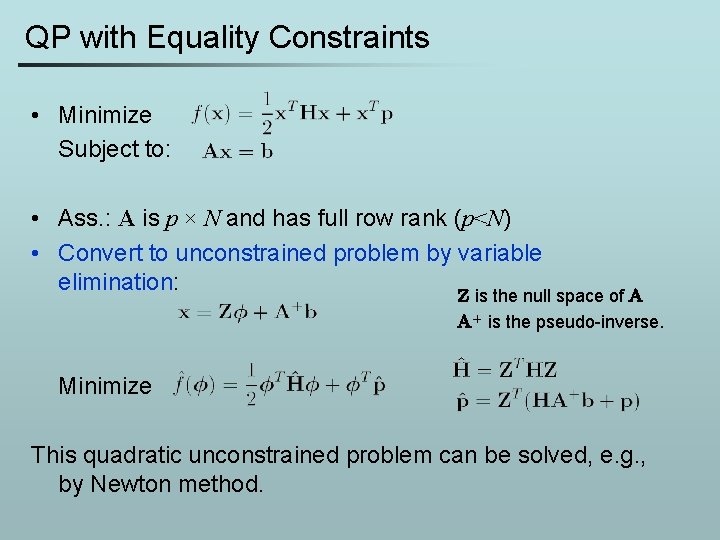QP with Equality Constraints • Minimize Subject to: • Ass. : A is p × N and has full row rank (p<N) • Convert to unconstrained problem by variable elimination: Z is the null space of A A+ is the pseudo-inverse. Minimize This quadratic unconstrained problem can be solved, e. g. , by Newton method.QP with inequality constraints • Minimize Subject to: • First we check if the unconstrained minimizer is feasible. If yes we are done. If not we know that the minimizer must be on the boundary and we proceed with an active-set method. • xk is the current feasible point • is the index set of active constraints at xk • Next iterate is given byActive-set method • How to find dk? – To remain active thus – The objective function at xk+d becomes where • The major step is a QP sub-problem subject to: • Two situations may occur: or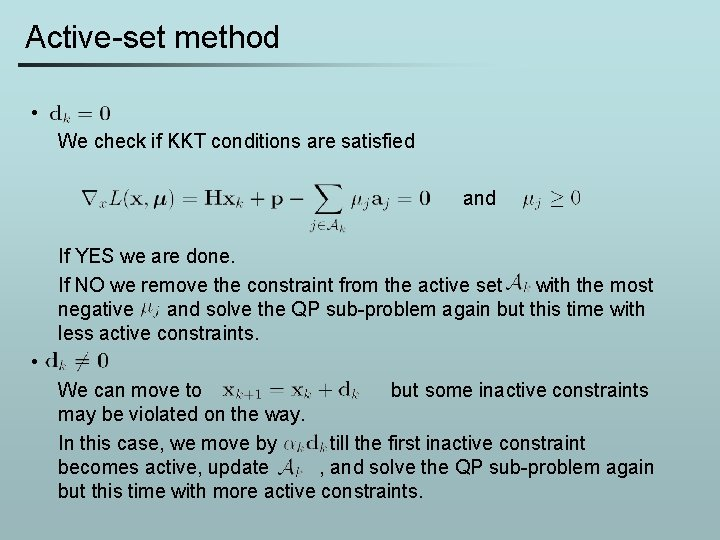Active-set method • We check if KKT conditions are satisfied and If YES we are done. If NO we remove the constraint from the active set with the most negative and solve the QP sub-problem again but this time with less active constraints. • We can move to but some inactive constraints may be violated on the way. In this case, we move by till the first inactive constraint becomes active, update , and solve the QP sub-problem again but this time with more active constraints.General Nonlinear Optimization • Minimize subject to: f(x) where the objective function and constraints are nonlinear. 1. 2. 3. 4. 5. For a given approximate Lagrangien by Taylor series → QP problem Solve QP → descent direction Perform line search in the direction → Update Lagrange multipliers → Repeat from Step 1.General Nonlinear Optimization Lagrangien At the kth iterate: and we want to compute a set of increments: First order approximation of and constraints: • • • These approximate KKT conditions corresponds to a QP program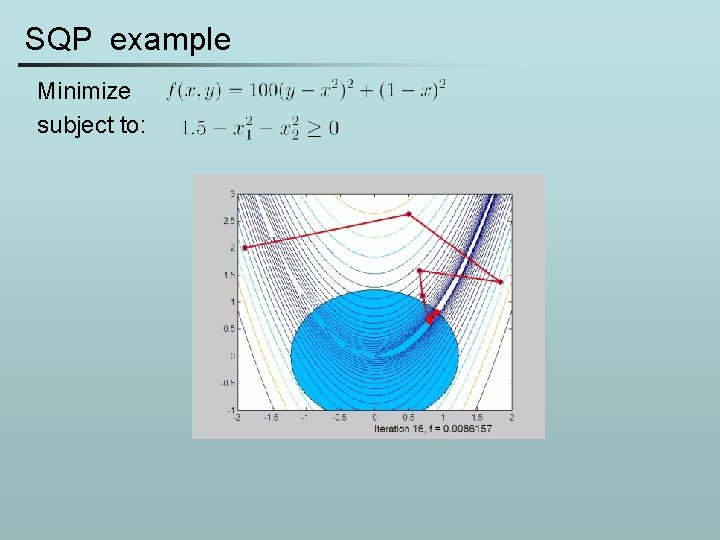SQP example Minimize subject to: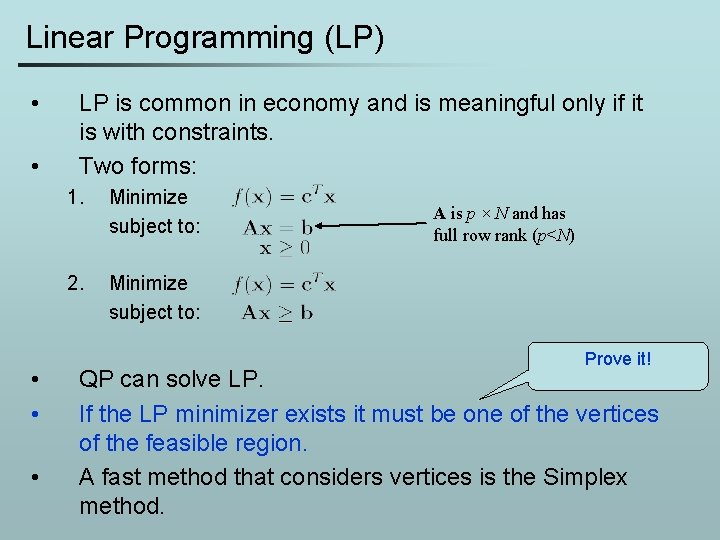Linear Programming (LP) • • • LP is common in economy and is meaningful only if it is with constraints. Two forms: 1. Minimize subject to: 2. Minimize subject to: A is p × N and has full row rank (p<N) Prove it! QP can solve LP. If the LP minimizer exists it must be one of the vertices of the feasible region. A fast method that considers vertices is the Simplex method.